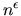Next: Robert Connelly - Holes Up: Discrete Geometry / Géométrie Previous: T. Bisztriczky - A

## Jin-Yi Cai - A new transference theorem in geometry of numbers with applications to Ajtai's connection factor

 JIN-YI CAI, Department of Computer Science, University of Buffalo, Buffalo, New York  14260, USA A new transference theorem in geometry of numbers with applications to Ajtai's connection factor

Geometry of Numbers was christened by Minkowski as a synthesis of geometric tools for number theoretic problems such as Diophantine approximations and quadratic forms.

Not only are they fascinating, lattice problems may provide a new class of public-key cryptographic protocols usable in any communication network, such as the internet and the World-Wide-Web. We will discuss a recent breakthrough of Ajtai on a connection of average-case and the worst-case complexity of lattice problems, and its implication for the design of provably secure public-key cryptography.

We prove a new transference theorem in the geometry of numbers, giving optimal bounds relating the successive minima of a lattice with the minimal length of generating vectors of its dual, improving previously the best transference theorem of this type due to Banaszczyk. We also prove a stronger bound for the special class of lattices possessing-unique shortest lattice vectors which play an important role in the Ajtai-Dwork Crypto-system.

The theorems imply consequent improvement of the Ajtai connection factors in the connection of average-case to worst-case complexity of the shortest lattice vector problem.Next: Robert Connelly - Holes Up: Discrete Geometry / Géométrie Previous: T. Bisztriczky - A